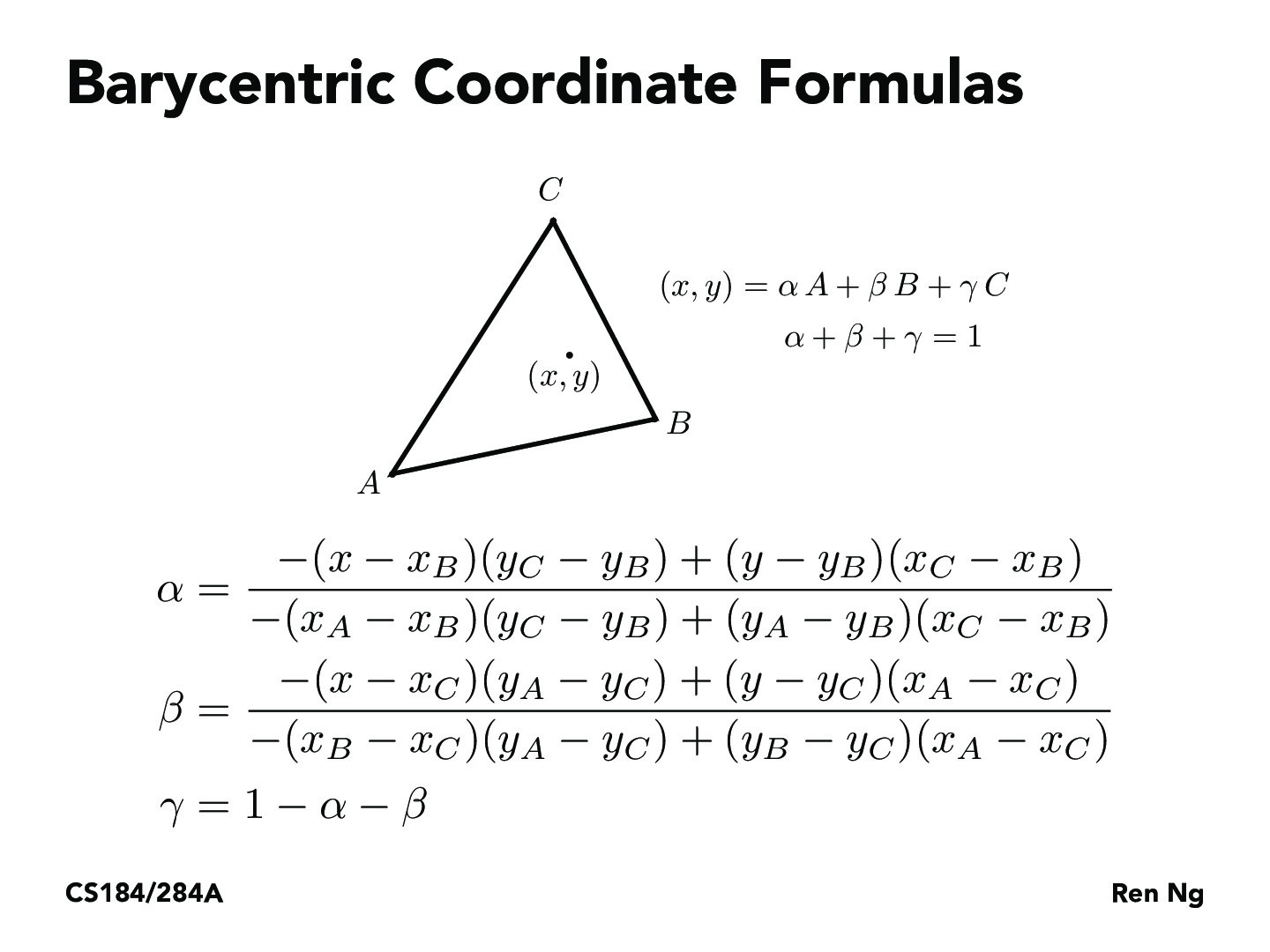Lecture 5: Texture Mapping (20)gavinmak

Could you use barycentric coordinates for point-in-triangle tests? If so, would this be faster than the 3 line tests?d00ble

The lecture seems to imply that we assume the point is already confirmed (via 3 line test) to be in the triangle before we calculate the barycentric coordinatesI don't see where it implies the point is confirmed inside the triangle (also see the first of these slides where it suggests these coordinates can be used for point-in-triangle tests).SuperMaltese

i feel like on average it might be faster, because the moment you know one coordinate is negative or greater than one, you can skip the rest of the operations. Whereas for the regular point in triangle, you have to test all three sides always.camrankolahdouz

Why can you not stop after the first failed for the regular line test?^andrewdotwang

It does seem like you could use barycentric coordinates as a point-in-triangle test, as if any of alpha/beta/gamma are less than 0 then the point is not in the triangle region.andrewdotwang

Another interesting application of barycentric coordinates as a test could be that if alpha, beta, gamma don't add up to 1, then we know that the point is not even in the plane spanned by the three triangle vertices.kalebblack

Barycentric coordinates can be generalized to higher dimensions by finding the inverse of a matrix having columns that are vertices of the simplex subtracted by one other vertex.

https://en.wikipedia.org/wiki/Barycentric_coordinate_system#Barycentric_coordinates_on_tetrahedra

You must be enrolled in the course to comment